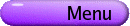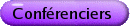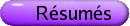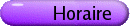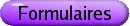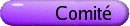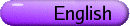Variables complexes Org: Thomas Bloom (Toronto) et Paul Gauthier (Montréal)[PDF] GAUTAM BHARALI, University of Michigan, Department of Mathematics, East Hall, Ann Arbor, MI 48109, USA Polynomial approximation, local polynomial convexity, and degenerate CR singularities [PDF] This talk will present a few advances on some earlier work on the following question: when is a smooth real surface S Ì C2 locally polynomially convex at a point p Î S? This question is complicated by the presence of points in the surface S that have complex tangents. Such points are called CR singularities. Let p Î S be a CR singularity at which the order of contact of the tangent plane with S is greater than 2; i.e., a degenerate CR singularity. We will discuss a sufficient condition for S to be locally polynomially convex at a degenerate CR singularity. In demonstrating this sufficient condition, we will need a new result about the uniform algebra on a closed disc in C generated by z and a complex-valued continuous function F. This result may be of independent interest because the function F here is allowed to be non-smooth; this result may thus be viewed as a Mergelyan-type theorem for complex-valued F. LEN BOS, University of Calgary, Calgary, Alberta Metrics Associated to Polynomial Inequalities [PDF] Suppose that K is a compact subset of Rn. We discuss a number of metrics on K that are closely related to Markov-Bernstein type inequalities on the derivatives of polynomials, as well as to the Siciak-Zahariuta extremal function for K as a subset of Cn. We also discuss some conjectured relations between these metrics and the distribution of extremal point sets such as Fekete points. ALEXANDER BRUDNYI, University of Calgary Holomorphic Functions of Slow Growth on Coverings of Pseudoconvex Domains in Stein Manifolds [PDF] We study holomorphic functions of slow growth on coverings of pseudoconvex domains in Stein manifolds. In particular, we extend and strengthen certain results of Gromov, Henkin and Shubin on holomorphic L2 functions on coverings of pseudoconvex manifolds in the case of coverings of Stein manifolds. DAN BURNS, University of Michigan, Ann Arbor, MI 48109, USA Exterior Monge-Ampère solutions for real convex bodies [PDF] We study solutions of the homogeneous complex Monge-Ampère equation (¶[`(¶)] u)n = 0 in Cn \K, where K is a compact convex set in Rn Ì Cn for a plurisubharmonic function growing logarithmically on Cn and tending to zero along K. The solution we are considering is therefore the Siciak-Zaharjuta extremal function associated to K. We use the method of characteristics to describe the solution. We describe a variational problem for Robin constants associated to holomorphic disks passing through the hyperplane at infinity in CPn, which is in a sense dual to the Kobayashi-Royden functional on disks used to define the infinitesimal Kobayashi distance. We use Lempert's work along these lines for the exteriors of strictly convex sets in Cn, passing to a limit of a sequence of approximating domains. The characteristic curves of the extremal function for the limit real convex set always exist and we show they are given by quadrics in CPn. The variational formulation and elementary geometry enable us to analyze the extremal functions fairly explicitly, especially under weak regularity conditions on K. An application is given to polynomial approximation in higher dimensions. This is joint work with Norman Levenberg (Indiana University) and Sione M'au (Aukland University). JOE CALLAGHAN, University of Toronto A Green's function for theta-incomplete polynomials [PDF] Let K be a compact subset of n-dimensional complex space and f a continous function on K. For 0 < q < 1, define a q-incomplete polynomial as any polynomial P with no terms of degree smaller than q times the degree of P. We seek necessary and sufficient conditions on f and K, for f to be the uniform limit on K, of q-incomplete polynomials. Our approach is to define an appropriate Green's function for q-incomplete polynomials. Then the solution to the approximation problem can be phrased in terms of the Monge-Ampere measure of this function. More precisely, this type of approximation will be possible exactly when f vanishes outside of the support of this measure. FRED CHAPMAN, Waterloo A Sufficient Condition for Uniform Convergence of a New Class of Newton Interpolation Series in Two Complex Variables [PDF] The name "Geddes series" refers to an extensive catalogue of new classes of series expansions for interpolating and approximating multivariate functions. The catalogue includes classes such as Geddes-Taylor series, Geddes-Fourier series, and a surprisingly large number of other classes. The general Geddes series scheme was invented by the presenter and named in honor of his thesis supervisor in 2003. The simplest class of Geddes series is the Geddes-Newton series, which interpolates a function of two variables on the lines of a two-dimensional grid; when either variable is held fixed, a Geddes-Newton series reduces to a generalized Newton interpolation series in the free variable. Grids with infinitely many lines generate Geddes-Newton series with infinitely many terms. We conjecture that if the original function is analytic in two complex variables over a sufficiently large region, the resulting Geddes-Newton series converges uniformly to the original function on every sufficiently small compact set containing all the grid lines. We also conjecture that the rate of convergence is linear or superlinear. This talk describes our progress to date in proving these conjectures. To that end, we will present a new contour integral remainder formula, new rigorous error estimates, and a new sufficient condition for uniform convergence at a linear or superliner rate. All that remains is to prove that this sufficient condition is always satisfied. We will also use Maple to present two applications which demonstrate the rapid convergence of Geddes-Newton series in practice: (1) the fast and accurate evaluation of certain kinds of multiple integrals in four real dimensions, and (2) the uniform approximation of special functions by elementary functions. This talk presents joint research with Keith Geddes. DAN COMAN, Department of Mathematics, Syracuse University, Syracuse, NY 13244-1150 Invariant currents and dynamical Lelong numbers [PDF] Let f be a polynomial automorphism of Ck of degree l, whose rational extension to Pk maps the hyperplane at infinity to a single point. Given any positive closed current S on Pk of bidegree (1,1), we show that the sequence l-n(fn)* S converges in the sense of currents on Pk to a linear combination of the Green current T+ of f and the current of integration along the hyperplane at infinity. We give an interpretation of the coefficients in terms of generalized Lelong numbers with respect to an invariant dynamical current for f-1. The results are joint work with Vincent Guedj. BRUCE GILLIGAN, University of Regina, Regina, SK S4S 0A2 Kaehler homogeneous manifolds [PDF] Let G be a connected complex Lie group and H a closed, complex subgroup of G. In this talk we will assume that the complex homogeneous manifold X : = G/H is Kähler. Kähler homogeneous manifolds X are completely understood if X is compact or the metric is G-invariant. The situation is also understood if the group G is semisimple, solvable, or a direct product S×R of its radical R with a maximal semisimple subgroup S of G. Attempts to construct examples of noncompact manifolds X homogeneous under a nontrivial semidirect product G = S \ltimes R with a not necessarily G-invariant Kähler metric motivated this work. In this setting the S-orbit S/SÇH in X is Kähler. Thus SÇH is an algebraic subgroup of S. The Kähler assumption on X ought to imply the S-action on the base Y of any homogeneous fibration X ® Y is algebraic too. Natural considerations allow a reduction to the case where H=G is a discrete subgroup and there is a homogeneous fibration X = G/G® G/I = : Y with I° an abelian, normal subgroup of G and the fiber I°/(I° ÇG) a Cousin group, i.e., a complex Lie group with no nonconstant holomorphic functions. We prove an algebraic condition does hold in the homogeneous manifold Y = [^(G)]/[^(G)], where [^(G)] : = G/I° and [^(G)] : = I/I°. Namely, we show that an element [^(g)] Î [^(G)] of infinite order lying in a semisimple subgroup [^(S)] of [^(G)] is an obstruction to the existence of a Kähler metric on X. So if X is Kähler, then [^(S)] Ç[^(G)] is finite. As a consequence, if the group [^(G)] is a linear algebraic group whose radical [^(R)] is a vector group and the representation of [^(S)] on [^(R)] is linear with no nonzero invariant vector, then G/G cannot be Kähler. An example of such a group [^(G)] is the affine group of Cn for n > 1. NIHAT GOGUS, Department of Mathematics, 215 Carnegie Hall, Syracuse University, Syracuse, NY 13244, USA Fusion and Localization of Plurisubharmonic Functions [PDF] We address the problem of continuity of plurisubharmonic envelopes. A bounded domain D is called c-regular if the plurisubharmonic envelope of every continuous function on [`(D)] extends continuously to [`(D)]. Using Gauthier's Fusion Lemma, we show that a domain is locally c-regular if and only if it is c-regular. IAN GRAHAM, University of Toronto, Toronto, ON The Caratheodory-Cartan-Kaup-Wu theorem on an infinite-dimensional Hilbert space [PDF] Let W be a bounded convex domain in a separable Hilbert space. Let f : W® W be a holomorphic mapping with a fixed point p. We give a criterion, in terms of triangularizability and spectral properties of dfp, for f to be biholomorphic. This is joint work with Joseph A. Cima (Chapel Hill), Kang-Tae Kim (Pohang University of Science and Technology, Korea), and Steven G. Krantz (Washington University, St. Louis). DANIEL JUPITER, Department of Mathematics, Texas A&M University, College Station, TX 77843-3368 Global Approximation of CR Functions on CR Manifolds [PDF] We discuss the problem of globally approximating CR functions on CR manifolds. Some known results in this direction will be mentioned, as will obstructions to solving the problem. We present a new result, joint with Al Boggess (Texas A&M): approximation on Bloom-Graham model graphs. FINNUR LARUSSON, University of Western Ontario, London, Ontario The Siciak-Zahariuta extremal function as the envelope of disc functionals [PDF] The Siciak-Zahariuta extremal function of a subset X of complex affine space, also known as the pluricomplex Green function with logarithmic growth at infinity, is the supremum of all entire plurisubharmonic functions of minimal growth that are negative on X. In the case when X is convex, there is a disc formula for the Siciak-Zahariuta extremal function due to Lempert. We will describe new joint work with Ragnar Sigurdsson in which Lempert's formula is generalized to arbitrary open sets. EUGENE POLETSKY, Syracuse University, Syracuse, NY 13244, USA Relative Disk Envelopes [PDF] Let X be a complex manifold, Y be an open subset of X and let f be an upper semicontunuous function on Y. Consider the space H(X,Y) of all analytic disks in X whose boundaries lie in Y. On this space we introduce an equivalence relation: two analytic disks are equivalent if their centers coincide and they can be connected by a continuous curve in H(X,Y). We show that on the set Y¢ of equivalence classes there is a local homeomorphism r into X that defines on Y¢ a structure of a complex manifold. We define the relative disk envelope of f on X as the infimum of the integrals of f over the boundaries of all analytic disks in H(X,Y) with centers at z0 Î X and boundaries in Y. As the result we get a function on Y¢ which is plurisubharmonic. This approach immediately generates many geometric questions that will be also discussed. RASUL SHAFIKOV, University of Western Ontario Uniformization of domains with spherical boundary [PDF] In this talk I will discuss the problem of uniformization of Stein domains with spherical boundaries. ZBIGNIEW SLODKOWSKI, University of Illinois at Chicago, Department of Math., Stat. and Comp. Sci., Chicago, IL 60302 Complex surfaces with real analytic plurisubharmonic exhaustion function [PDF] This paper is a continuation of the earlier work of the authors, Minimal kernels of a class of weakly complete spaces (J. Funct. Anal. 210(2004), 125-147). A minimal kernel (defined in that paper) of a weakly complete manifold was shown to be a union of a family of pairwise disjoint compact pseudoconcave sets. If the manifold X admits a real analytic exhaustion function and has complex dimension two, then either the kernel is empty (and X is Stein), or the kernel is the union of an isolated sequence of compact complex curves (and X can be obtained by blow-ups of a Stein space), or the kernel is equal to X, which has to be foliated (in a relaxed sense of the word) by compact complex curves and/or compact Levi flat hypersurfaces (with possible singularities). The details of this structure are most transparent when the exhaustion function has only isolated critical points. This is joint work with Giuseppe Tomassini.